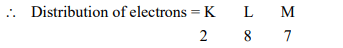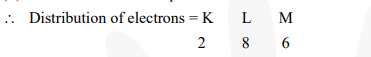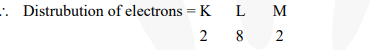# How will you find the valency of chlorine, sulphur and magnesium?

Question.
How will you find the valency of chlorine, sulphur and magnesium?

Solution:

(i) Atomic number of chlorine $=17$Thus, outermost shell has 7 electrons. It can easily complete its octet by gaining one electron. Hence, its valency = 1.

(ii) Atomic number of sulphur = 16Thus, outermost shell has 6 electrons.

Hence, valency = 8 – 6 = 2

(iii) Atomic number of magnesium = 12\Thus, outermost shell has 2 electrons. It can easily complete its octet by loosing two electrons. Hence, its valency = 2.